Working time - shortening

The media often speculates about the change (especially to shorten) the working time of the five-day eight-hour working week to another working model. Calculate how many hours a day the employee would have to work a day at 3-day work week if he must work the same number of hours weekly.

Result

h = 13:20 hSolution:Leave us a comment of this math problem and its solution (i.e. if it is still somewhat unclear...):Be the first to comment!To solve this verbal math problem are needed these knowledge from mathematics:

Do you have a linear equation or system of equations and looking for its solution? Or do you have quadratic equation?

Next similar math problems:

1. Father and son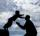Father is 44 years old, his son 16 years. Determine how many years ago was the father five times older than the son.
2. Pump680 liters of water were pumped in 8 minutes. How many liters was spent in 56 minutes?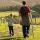Daddy is a 46 year old and son is at age of 16. When (which year) was/will daddy 5 times more years than his son?
4. Pills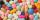If it takes 20 minutes to run a batch of 100 pills how many minutes would it take to run a batch of 50 pills
5. Minutes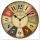Determine the difference in minutes: T1 = 2 3/20 h T2 = 2.3 h
6. TimeageSeven times of my age is 8 less than the largest two-digit number. How old I am?
7. Jan and DanJan and Dan had the same money. Jan bought 5 workbooks and left him 15 CZK. Dan 6 and left him nothing. How much money have in total?
8. Seven timesWhich number seven times is just as higher as 27, how much is smaller than 29?
9. NumberDetermine unknown number if you know that difference between five times and triple of number is 42.
10. Six te 2If 3t-7=5t, then 6t=
11. Forest nurseryIn the forest nursery after winter, they found that 1/10 stems died out of them. For them, they land 193 new spruces. How many spruces are in the forest nursery?
12. If-then equationIf 5x - 17 = -x + 7, then x =
13. Eq1Solve equation: 4(a-3)=3(2a-5)
14. Addition of Roman numbersAdded together and write as decimal number: LXVII + MLXIV
15. Simple equation 8Solve the following equation: 36=-(1+7x)-6(-7-x)
16. PopsiclesFrancis went to buy ice lollies. If he buy 8 popsicles he missed 4 USD. When he buy 7 popsicles, got back 1 USD. How many USD was a popsicle?
17. Jam cakes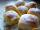Mom baked a third of plum jam cakes, one third cheesecakes and 18 poppy. How many cakes she had bake?